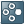Show TOC

###Time-Shift InstructionsTo simplify the calculation of leads and lags in financial reporting applications, the following instructions are available for SQL-based logic:

• PRIOR

• NEXT

• BASE

• FIRST

The instructions PRIOR and NEXT support an optional numeric parameter. This parameter represents the number of time periods by which the current period must be shifted. If omitted, the function assumes a time shift of one period (forward or backwards). Negative values are accepted (a negative value for a NEXT function corresponds to a positive value for a PRIOR function, and a positive value for a PRIOR function corresponds to a negative value for a NEXT function).Example

`TIME=NEXT // In a monthly application this means next month`

`TIME=PRIOR(3) // Three periods backwards`

`TIME=NEXT(-3) // Same as PRIOR(3)`

End of the example.

The keyword BASE always represents the last period of the prior fiscal year. When the fiscal year is a normal calendar year and the frequency is monthly, the base period of 2004.JUN is 2003.DEC.

The instruction BASE can be useful in YTD applications, where the opening balances need to be retrieved from the last period of the prior year.

The keyword FIRST always represents the first period of the current fiscal year. When the fiscal year is a typical calendar year and the frequency is monthly, the base period of 2004.JUN is 2004.JAN. In case the time shift goes past the boundaries of the TIME dimension, these time shift functions return no period.

These functions can be used in four ways:

1. To redirect the destination period in a *REC statement

Example 1: `*REC(TIME=NEXT)`

Example 2: `*REC(TIME=BASE)`

2. To retrieve a value from a different period in a *REC statement

Example 1: `*REC(FACTOR=GET(TIME=PRIOR(3))`

Example 2: `*REC(FACTOR=GET(TIME=BASE)`

3. To add periods to the selected data region in a XDIM_MEMBERSET statement

Example: `*XDIM_MEMBERSET TIME=PRIOR, %TIME_SET%`

In this example, if the first modified period is 2004.APR, the instruction PRIOR adds 2004.MAR to the region to process.

4. When the keywords PRIOR, FIRST or BASE are added to a XDIM_MEMBERSET instruction, the time period PRIOR, FIRST or BASE can be also evaluated in a WHEN / ENDWHEN structure, as in the following example:

`*WHEN TIME`

`*IS PRIOR`

`// ignore`

`*ELSE`

`*REC(…)`

`*ENDWNHEN`

In the presence of an XDIM_MEMBERSET containing the PRIOR keyword, like in the above example, the WHEN structure recognizes 2004.MAR as PRIOR period.